# windows technique psp.pptx

1 de Apr de 2023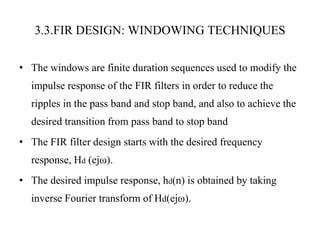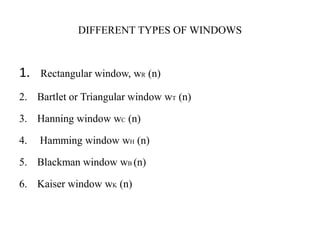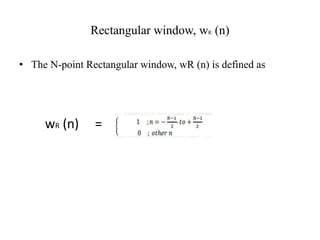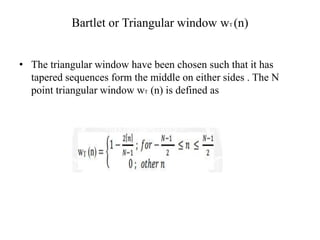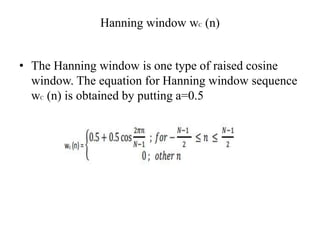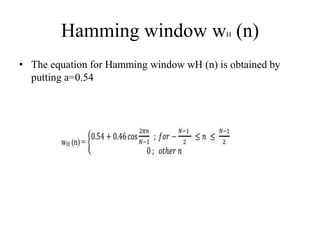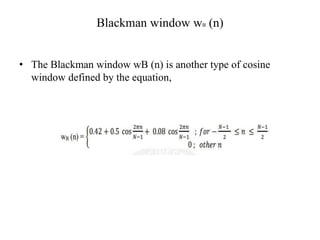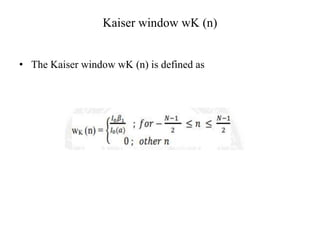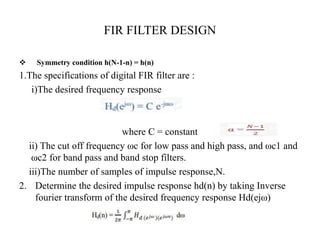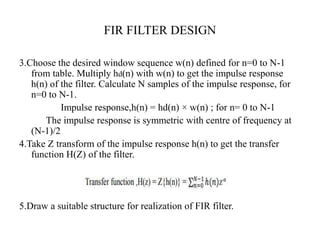1 de 12

### windows technique psp.pptx

1. WINDOWS TECHNIQUE Presented By Miss . D. Saiprasanthi Assistant Professor E.E.E. Department
2. 3.3.FIR DESIGN: WINDOWING TECHNIQUES • The windows are finite duration sequences used to modify the impulse response of the FIR filters in order to reduce the ripples in the pass band and stop band, and also to achieve the desired transition from pass band to stop band • The FIR filter design starts with the desired frequency response, Hd (ejω). • The desired impulse response, hd(n) is obtained by taking inverse Fourier transform of Hd(ejω).
3. DIFFERENT TYPES OF WINDOWS 1. Rectangular window, wR (n) 2. Bartlet or Triangular window wT (n) 3. Hanning window wC (n) 4. Hamming window wH (n) 5. Blackman window wB (n) 6. Kaiser window wK (n)
4. Rectangular window, wR (n) • The N-point Rectangular window, wR (n) is defined as wR (n) =
5. Bartlet or Triangular window wT (n) • The triangular window have been chosen such that it has tapered sequences form the middle on either sides . The N point triangular window wT (n) is defined as
6. Hanning window wC (n) • The Hanning window is one type of raised cosine window. The equation for Hanning window sequence wC (n) is obtained by putting a=0.5
7. Hamming window wH (n) • The equation for Hamming window wH (n) is obtained by putting a=0.54
8. Blackman window wB (n) • The Blackman window wB (n) is another type of cosine window defined by the equation,
9. Kaiser window wK (n) • The Kaiser window wK (n) is defined as
10. FIR FILTER DESIGN  Symmetry condition h(N-1-n) = h(n) 1.The specifications of digital FIR filter are : i)The desired frequency response where C = constant ii) The cut off frequency ωc for low pass and high pass, and ωc1 and ωc2 for band pass and band stop filters. iii)The number of samples of impulse response,N. 2. Determine the desired impulse response hd(n) by taking Inverse fourier transform of the desired frequency response Hd(ejω)
11. FIR FILTER DESIGN 3.Choose the desired window sequence w(n) defined for n=0 to N-1 from table. Multiply hd(n) with w(n) to get the impulse response h(n) of the filter. Calculate N samples of the impulse response, for n=0 to N-1. Impulse response,h(n) = hd(n) × w(n) ; for n= 0 to N-1 The impulse response is symmetric with centre of frequency at (N-1)/2 4.Take Z transform of the impulse response h(n) to get the transfer function H(Z) of the filter. 5.Draw a suitable structure for realization of FIR filter.
12. THANKYOU